2021 NFL Defensive Player of the Year Odds
+200
2 to 1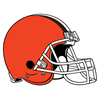Myles Garrett
24.1% implied probability

+500
5 to 1Trevon Diggs
12.1% implied probability

+700
7 to 1T.J. Watt
9.0% implied probability

+800
8 to 1Matthew Judon
8.0% implied probability

+1200
12 to 1Aaron Donald
5.6% implied probability

+1800
18 to 1J.C. Jackson
3.8% implied probability

+2500
25 to 1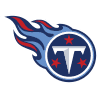Kevin Byard
2.8% implied probability

+3500
35 to 1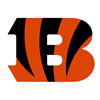Trey Hendrickson
2.0% implied probability

+3500
35 to 1Harold Landry
2.0% implied probability

+3500
35 to 1Chandler Jones
2.0% implied probability

+3500
35 to 1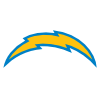Derwin James
2.0% implied probability

+3500
35 to 1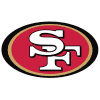Nick Bosa
2.0% implied probability

+4000
40 to 1Von Miller
1.8% implied probability

+4000
40 to 1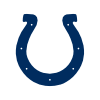Darius Leonard
1.8% implied probability

+4000
40 to 1Jalen Ramsey
1.8% implied probability

+4000
40 to 1Joey Bosa
1.8% implied probability

+5000
50 to 1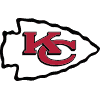Chris Jones
1.4% implied probability

+5000
50 to 1Devin White
1.4% implied probability

+5000
50 to 1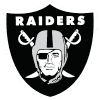Maxx Crosby
1.4% implied probability

+5000
50 to 1Brian Burns
1.4% implied probability

+5000
50 to 1Markus Golden
1.4% implied probability

+6500
65 to 1Jessie Bates
1.1% implied probability

+6500
65 to 1Haason Reddick
1.1% implied probability

+8000
80 to 1Devin Bush
0.9% implied probability

+8000
80 to 1Josh Allen
0.9% implied probability

+10000
100 to 1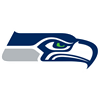Bobby Wagner
0.7% implied probability

+10000
100 to 1Justin Simmons
0.7% implied probability

+10000
100 to 1Budda Baker
0.7% implied probability

+10000
100 to 1Patrick Queen
0.7% implied probability

+10000
100 to 1L'Jarius Sneed
0.7% implied probability

+10000
100 to 1Jamal Adams
0.7% implied probability

+10000
100 to 1Dont'a Hightower
0.7% implied probability

+10000
100 to 1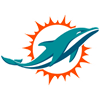Xavien Howard
0.7% implied probability

+10000
100 to 1DeMarcus Lawrence
0.7% implied probability